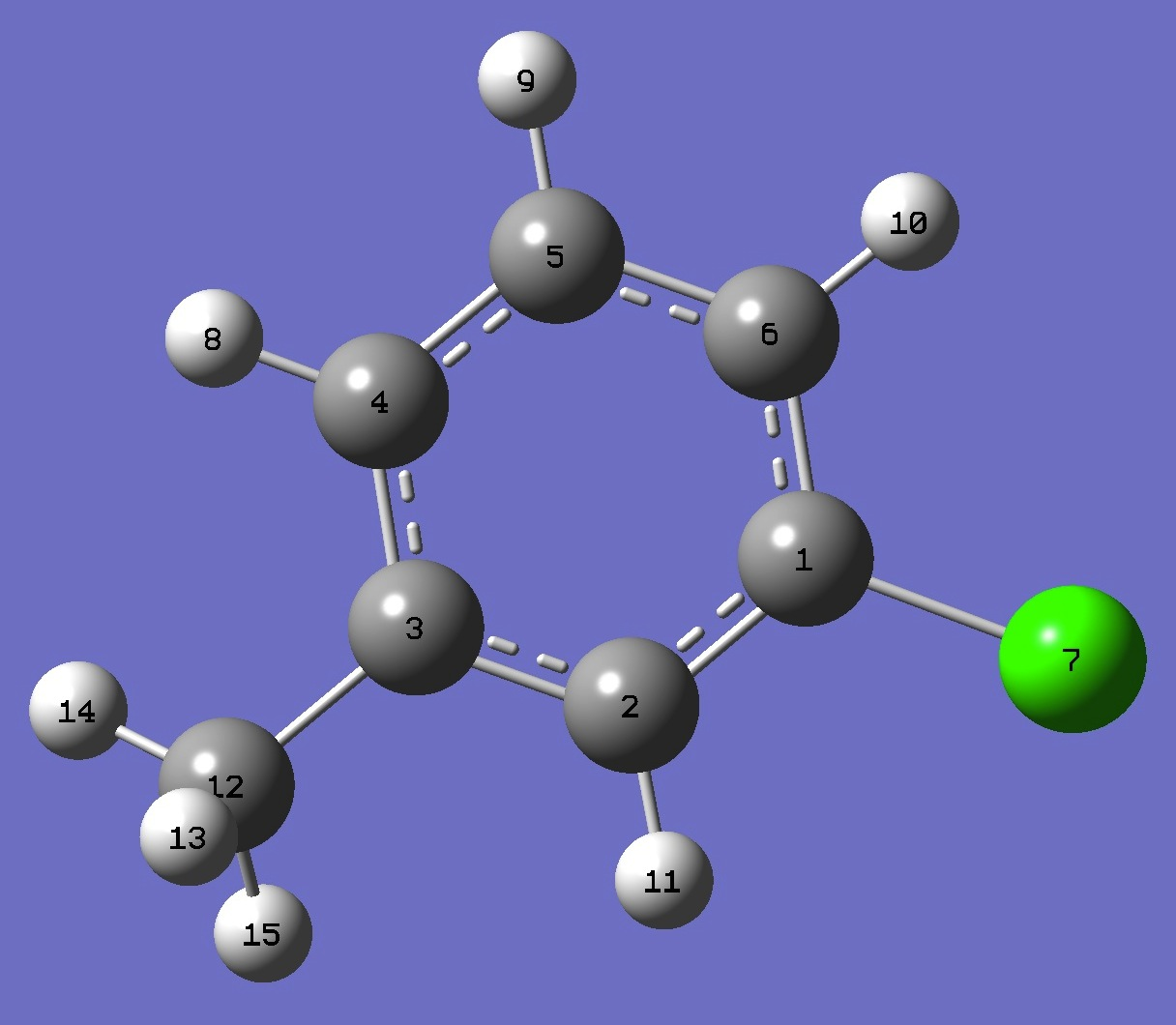m-Cl-C6H4-CH3

Chlorine

in meta-Chlorotoluene

Complete chlorine nqcc tensors have been determined by Nair et al. .

The nqcc's were calculated here on a structure determined by B3P86/6-31G(3d,3p) optimization.  These calculated nqcc's are compared with the experimental values in Table 1.  Structure parameters (in Z-matrix format) are given in Table 2, rotational constants in Table 3.

In Table 1, subscripts a,b,c refer to the principal axes of the inertia tensor, subscripts x,y,z to the principal axes of the nqcc tensor.  Ø (degrees) is the angle between its subscripted parameters.  ETA = (Xxx - Xyy)/Xzz.

RMS is the root mean square difference between calculated and experimental diagonal nqcc's (percentage of the average of the magnitudes of the experimental diagonal nqcc's).  RSD is the calibration residual standard deviation for the B1LYP/TZV(3df,2p) model for calculation of the nqcc's.

 Table 1.  Chlorine nqcc's in meta-Chlorotoluene (MHz).  Calculation was made on the B3P86/6-31G(3d,3p) ropt structure. Calc. Expt.  * 35Cl Xaa - 60.76 - 60.325(97) Xbb 28.00 27.372(77) ** Xcc 32.76 32.953(77) ** Xab 32.82 32.48(63) Xac 0.03 Xbc 0.01 RMS 0.46 (1.1 %) RSD 0.49 (1.1 %) Xxx 38.82 38.090 Xyy 32.76 32.953 Xzz - 71.58 - 71.044 ETA - 0.0847 Øz,a 18.24 Øa,CCl 18.25 Øz,CCl 0.01 37Cl Xaa - 48.30 - 47.913(85) Xbb 22.48 21.933(63) *** Xcc 25.82 25.920(63) *** Xab 25.31 24.84(64) Xac 0.00 Xbc 0.00 RMS 0.36 (1.1 %) RSD 0.44 (1.1 %)

* Obtained from XIAM fit in Table 2 of Ref. .

** Calculated here from Xaa and Xbb -  Xcc = -5.58(12) MHz.

*** Calculated here from Xaa and Xbb -  Xcc = -3.927(93) MHz.

 Table 2.  meta-Chlorotoluene:  B3P86/6-31G(3d,3p) ropt structure parameters (Å and degrees).C  C,1,B1  C,2,B2,1,A1  C,3,B3,2,A2,1,D1,0  C,4,B4,3,A3,2,D2,0  C,5,B5,4,A4,3,D3,0  Cl,1,B6,6,A5,5,D4,0  H,4,B7,3,A6,2,D5,0  H,5,B8,4,A7,3,D6,0  H,6,B9,5,A8,4,D7,0  H,2,B10,1,A9,6,D8,0  C,3,B11,2,A10,1,D9,0  H,12,B12,3,A11,2,D10,0  H,12,B13,3,A12,2,D11,0  H,12,B14,3,A13,2,D12,0 B1=1.38808094  B2=1.39535355  B3=1.39506466  B4=1.38948093  B5=1.38890835  B6=1.73754697  B7=1.08551363  B8=1.08490273  B9=1.08305304  B10=1.08450051  B11=1.50238543  B12=1.09507184  B13=1.0919962  B14=1.09243101  A1=120.01881711  A2=118.6224513  A3=120.81167704  A4=120.63197122  A5=119.33382153  A6=119.40843638 A7=119.95306146  A8=121.42975745  A9=119.51614246  A10=120.3155239  A11=110.95337923  A12=111.25867639  A13=111.46526519  D1=0.24743207  D2=-0.24398838  D3=0.09148989  D4=179.7536431  D5=179.62555595  D6=179.88226168  D7=179.79749516  D8=179.78631052  D9=-178.67539917  D10=84.08328485  D11=-156.32451438  D12=-35.45765264

 Table 3.  meta-Chlorotoluene:  35Cl Species rotational constants (MHz), ropt = B3P86/6-31G(3d,3p). ropt Expt  A 3353. 3334.3188(40) B 1195. 1191.3515(11) C 886. 882.50133(59)

 K.P.R.Nair, S.Herbers, A.Lesarri, and J.-U. Grabow, J.Mol.Spectrosc. 361,1(2019).

p-Chlorotolueneo-Chlorotoluene
Chlorobenzene

1,3-Chlorofluorobenzene 1,3-Dichlorobenzene

1,4-Chlorofluorobenzene 1,4-Dichlorobenzene

1,2-Chlorofluorobenzene 1,2-Dichlorobenzene

cis-2-Chlorophenol trans-2-Chlorophenol

Molecules/Chlorine

3ClToluene.html